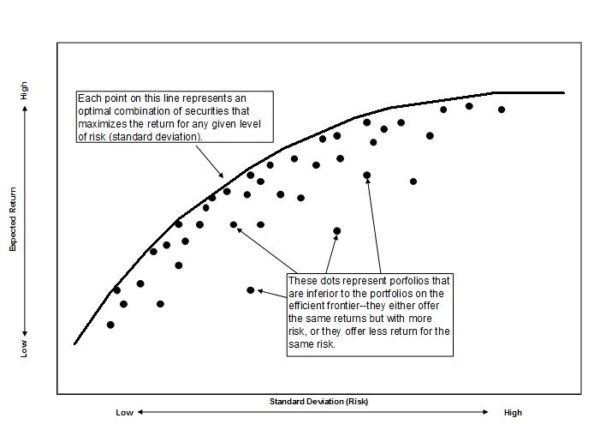## What is a Markowitz Efficient Set?

The Markowitz efficient set, also called the efficient frontier, is a mathematical concept that reflects the combinations or portfolios that generate the maximum expected return for various levels of risk.

In 1952, Harry Markowitz set the efficient frontier idea in motion when he published a formal portfolio selection model in The Journal of Finance. Markowitz continued to develop and publish research on the subject over the next 20 years, and other financial theorists contributed to the work. Markowitz won the 1990 Nobel Prize in Economics for his work on the efficient frontier and for related contributions to modern portfolio theory.

## How Does a Markowitz Efficient Set Work?

Different combinations of securities produce different levels of return. The efficient frontier represents the best of these combinations -- those that produce the maximum expected return for a given level of risk. The efficient set is the result of an evaluation of the expected returns, standard deviation and the covariances of a set of securities.

An example appears below. Note how the Markowitz efficient set allows investors to understand how a portfolio’s expected returns vary with the amount of risk (standard deviation) taken.The relationship securities have with each other is an important part of the Markowitz efficient set. Some security prices head the same direction under similar circumstances, but many securities zig when others zag. The more out of sync the securities in the portfolio are (that is, the lower their covariance), the smaller the risk (standard deviation) of the portfolio that combines them. This is why the efficient frontier is curved rather than linear--diversification makes the risk (standard deviation) of the portfolio lower than the risk (standard deviation) of each individual security.

Although diversified portfolios usually compose the points on the efficient frontier, sometimes the Markowitz efficient set includes portfolios that consist of a single security if it is the only way the investor can obtain the desired return with the least amount of risk.

## Why Does a Markowitz Efficient Set Matter?

When Markowitz introduced the Markowitz efficient set and the efficient frontier, it was groundbreaking in many respects. One of its largest contributions was its clear demonstration of the power of diversification.

Investors tend to choose, directly or indirectly, portfolios that generate the largest possible returns with the least amount of risk -- in other words, they tend to seek portfolios on the efficient frontier. However, there is no one Markowitz efficient set, because portfolio managers and investors can edit the number and characteristics of the securities in the investing universe to conform to specific needs. For example, a client may require the portfolio to have a minimum dividend yield, or the client may rule out investments in ethically or politically undesirable industries. Only the remaining securities are included in the calculations.

When he accepted the Nobel Prize, Markowitz said, “The existence of uncertainty is essential to the analysis of rational investment behavior.” Ironically, Markowitz’s effort to actually compute the investor’s optimal portfolio proves to be one of the most controversial aspects of the efficient frontier. Many argue that it reduces investment management to an algorithm involving nothing more than statistical relationships among securities rather than analysis of the underlying companies’ fundamental and financial characteristics.